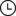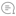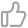# 【深度学习】图文并茂！用Keras LSTM构建编码器-解码器模型机器学习初学者 |742020-11-24 06:03000

### 实现

#### 1-预处理

``import stringimport numpy as npfrom keras.preprocessing.text import Tokenizerfrom keras.preprocessing.sequence import pad_sequencesfrom keras.models import Modelfrom keras.layers import LSTM, Input, TimeDistributed, Dense, Activation, RepeatVector, Embeddingfrom keras.optimizers import Adamfrom keras.losses import sparse_categorical_crossentropy# 翻译文件的路径path_to_data = 'data/spa.txt'# 读文件translation_file = open(path_to_data,"r", encoding='utf-8') raw_data = translation_file.read()translation_file.close()# 解析数据raw_data = raw_data.split('\n')pairs = [sentence.split('\t') for sentence in  raw_data]pairs = pairs[1000:20000]``

``def clean_sentence(sentence):    # 把这个句子小写    lower_case_sent = sentence.lower()    # 删除标点    string_punctuation = string.punctuation + "¡" + '¿'    clean_sentence = lower_case_sent.translate(str.maketrans('', '', string_punctuation))       return clean_sentence``

``def tokenize(sentences):    # 创建 tokenizer    text_tokenizer = Tokenizer()    # 应用到文本上    text_tokenizer.fit_on_texts(sentences)    return text_tokenizer.texts_to_sequences(sentences), text_tokenizer``

``# 清理句子english_sentences = [clean_sentence(pair) for pair in pairs]spanish_sentences = [clean_sentence(pair) for pair in pairs]# 标识化单词spa_text_tokenized, spa_text_tokenizer = tokenize(spanish_sentences)eng_text_tokenized, eng_text_tokenizer = tokenize(english_sentences)print('Maximum length spanish sentence: {}'.format(len(max(spa_text_tokenized,key=len))))print('Maximum length english sentence: {}'.format(len(max(eng_text_tokenized,key=len))))# 检查长度spanish_vocab = len(spa_text_tokenizer.word_index) + 1english_vocab = len(eng_text_tokenizer.word_index) + 1print("Spanish vocabulary is of {} unique words".format(spanish_vocab))print("English vocabulary is of {} unique words".format(english_vocab))``

``max_spanish_len = int(len(max(spa_text_tokenized,key=len)))max_english_len = int(len(max(eng_text_tokenized,key=len)))spa_pad_sentence = pad_sequences(spa_text_tokenized, max_spanish_len, padding = "post")eng_pad_sentence = pad_sequences(eng_text_tokenized, max_english_len, padding = "post")# 重塑spa_pad_sentence = spa_pad_sentence.reshape(*spa_pad_sentence.shape, 1)eng_pad_sentence = eng_pad_sentence.reshape(*eng_pad_sentence.shape, 1)``

#### 2.模型开发

##### 2.1-编码器

``input_sequence = Input(shape=(max_spanish_len,))embedding = Embedding(input_dim=spanish_vocab, output_dim=128,)(input_sequence)``

``input_sequence = Input(shape=(max_spanish_len,))embedding = Embedding(input_dim=spanish_vocab, output_dim=128,)(input_sequence)encoder = LSTM(64, return_sequences=False)(embedding)``

##### 2.2-解码器

``input_sequence = Input(shape=(max_spanish_len,))embedding = Embedding(input_dim=spanish_vocab, output_dim=128,)(input_sequence)encoder = LSTM(64, return_sequences=False)(embedding)r_vec = RepeatVector(max_english_len)(encoder)``

``input_sequence = Input(shape=(max_spanish_len,))embedding = Embedding(input_dim=spanish_vocab, output_dim=128,)(input_sequence)encoder = LSTM(64, return_sequences=False)(embedding)r_vec = RepeatVector(max_english_len)(encoder)decoder = LSTM(64, return_sequences=True, dropout=0.2)(r_vec)``

``input_sequence = Input(shape=(max_spanish_len,))embedding = Embedding(input_dim=spanish_vocab, output_dim=128,)(input_sequence)encoder = LSTM(64, return_sequences=False)(embedding)r_vec = RepeatVector(max_english_len)(encoder)decoder = LSTM(64, return_sequences=True, dropout=0.2)(r_vec)logits = TimeDistributed(Dense(english_vocab))(decoder)``

``enc_dec_model = Model(input_sequence, Activation('softmax')(logits))enc_dec_model.compile(loss=sparse_categorical_crossentropy,              optimizer=Adam(1e-3),              metrics=['accuracy'])enc_dec_model.summary()``

``model_results = enc_dec_model.fit(spa_pad_sentence, eng_pad_sentence, batch_size=30, epochs=100)``

``def logits_to_sentence(logits, tokenizer):    index_to_words = {idx: word for word, idx in tokenizer.word_index.items()}    index_to_words = ''     return ' '.join([index_to_words[prediction] for prediction in np.argmax(logits, 1)])index = 14print("The english sentence is: {}".format(english_sentences[index]))print("The spanish sentence is: {}".format(spanish_sentences[index]))print('The predicted sentence is :')print(logits_to_sentence(enc_dec_model.predict(spa_pad_sentence[index:index+1]), eng_text_tokenizer))``

### 结论

#### 附录：不使用重复向量的编解码器

`往期精彩回顾适合初学者入门人工智能的路线及资料下载机器学习及深度学习笔记等资料打印机器学习在线手册深度学习笔记专辑《统计学习方法》的代码复现专辑AI基础下载机器学习的数学基础专辑获取本站知识星球优惠券，复制链接直接打开：https://t.zsxq.com/y7uvZF6本站qq群704220115。加入微信群请扫码：`00

暂无评论~~
Ctrl+Enter